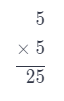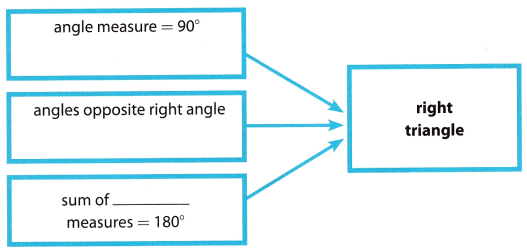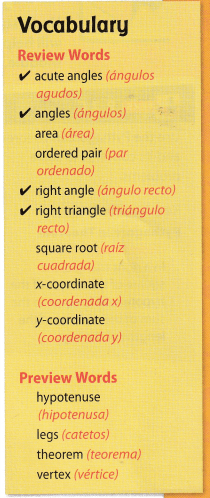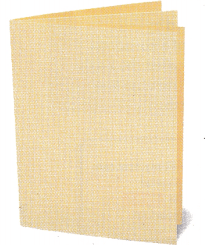# Texas Go Math Grade 8 Module 8 Answer Key The Pythagorean Theorem

Refer to our Texas Go Math Grade 8 Answer Key Pdf to score good marks in the exams. Test yourself by practicing the problems from Texas Go Math Grade 8 Module 8 Answer Key The Pythagorean Theorem.

## Texas Go Math Grade 8 Module 8 Answer Key The Pythagorean Theorem

Find the square of each number.

Question 1.
5 _________
To determine the square of a number x, denoted by x2 just multiply the given number x by itself.
Now, let us get the square of 5 or the equivalent of 52, just multiply 5 by itself, that is
52 = 5 × 5
= 25
or16 __________
When solving for the square of a number, we need to multiply the certain number by itself.
To find the square of 16, we simply multiply it by itself
162 = 16 × 16
= 256

Question 3.
-11 _________
To determine the square of a number x, denoted by x2 just multiply the given number x by itself.
Now, let us get the square of -11 or the equivalent of (-11)2, just multiply 11 by itself, that is
(-11)2 = -11(-11)
= 121

Question 4.
$$\frac{2}{7}$$ __________
To solve for the square of $$\frac{2}{7}$$, we simply multiply it by itself. Take note that in multiplying fractions, the product is equal to the product of all numerators divided by the product of all denominators.
($$\frac{2}{7}$$)2 = $$\frac{2}{7}$$ × $$\frac{2}{7}$$ = $$\frac{4}{49}$$

Evaluate each expression.

$$\sqrt{(6+2)^{2}+(3+3)^{2}}$$ __________
$$\sqrt{(6+2)^{2}+(3+3)^{2}}=\sqrt{8^{2}+6^{2}}$$ (First, operate within parentheses.) …… (1)
= $$\sqrt{64+36}$$ (Next, simplify exponents.) ………. (2)
= $$\sqrt{100}$$ (Then add and subtract left to right.) ……….. (3)
= 10 (Finally, take the square root.) ……… (4)
= 10

Question 6.
$$\sqrt{(9-4)^{2}+(5+7)^{2}}$$ __________
$$\sqrt{(9-4)^{2}+(5+7)^{2}}=\sqrt{5^{2}+12^{2}}$$ (First, operate within parentheses.) …… (1)
= $$\sqrt{25+144}$$ (Next, simplify exponents.) ………. (2)
= $$\sqrt{169}$$ (Then add and subtract left to right.) ……….. (3)
= 13 (Finally, take the square root.) ……… (4)
= 13

Question 7.
$$\sqrt{(10-6)^{2}+(15-12)^{2}}$$ __________
$$\sqrt{(10-6)^{2}+(15-12)^{2}}=\sqrt{4^{2}+3^{2}}$$ (First, operate within parentheses.) …… (1)
= $$\sqrt{16+9}$$ (Next, simplify exponents.) ………. (2)
= $$\sqrt{25}$$ (Then add and subtract left to right.) ……….. (3)
= 5 (Finally, take the square root.) ……… (4)
= 5

Question 8.
$$\sqrt{(6+9)^{2}+(10-2)^{2}}$$ __________
$$\sqrt{(6+9)^{2}+(10-2)^{2}}=\sqrt{15^{2}+8^{2}}$$ (First, operate within parentheses.) …… (1)
= $$\sqrt{225+64}$$ (Next, simplify exponents.) ………. (2)
= $$\sqrt{289}$$ (Then add and subtract left to right.) ……….. (3)
= 17 (Finally, take the square root.) ……… (4)
= 17

Simplify each expression.

Module 8 The Pythagorean Theorem Answer Key Question 9.
5(8)(10) ___________
5(8)(10) = (40)(10) (Multiply from left to right)
= 400

Question 10.
$$\frac{1}{2}$$(6)(12) ___________
$$\frac{1}{2}$$(6)(12) = (3)(12) (Multiply from left to right)
= 36

Question 11.
$$\frac{1}{3}$$(3)(12) ___________
$$\frac{1}{3}$$(3)(12) = (1)(12) (Multiply from left to right)
= 12

Question 12.
$$\frac{1}{2}$$(82)(4) ___________
Simplify expression
$$\frac{1}{2}$$(82)(4) = $$\frac{1}{2}$$(64)(4) (Simplify the exponent) …………. (1)
= (32)(4) (Multiply from left to right) ……………… (2)
= 128

Question 13.
$$\frac{1}{4}$$(10)2(15) ___________
Simplify expression
$$\frac{1}{4}$$(102)(15) = $$\frac{1}{4}$$(100)(15) (Simplify the exponent) …………. (1)
= (25)(15) (Multiply from left to right) ……………… (2)
= 375

Texas Go Math Grade 8 Module 8 Answer Key Pdf Question 14.
$$\frac{1}{3}$$(9)2(6) ___________
Simplify expression
$$\frac{1}{3}$$(92)(6) = $$\frac{1}{3}$$(81)(6) (Simplify the exponent) …………. (1)
= (27)(6) (Multiply from left to right) ……………… (2)
= 162

Visualize Vocabulary

Use the ✓ words to complete the graphic.Understand Vocabulary

Match the term on the left to the correct expression on the right.